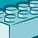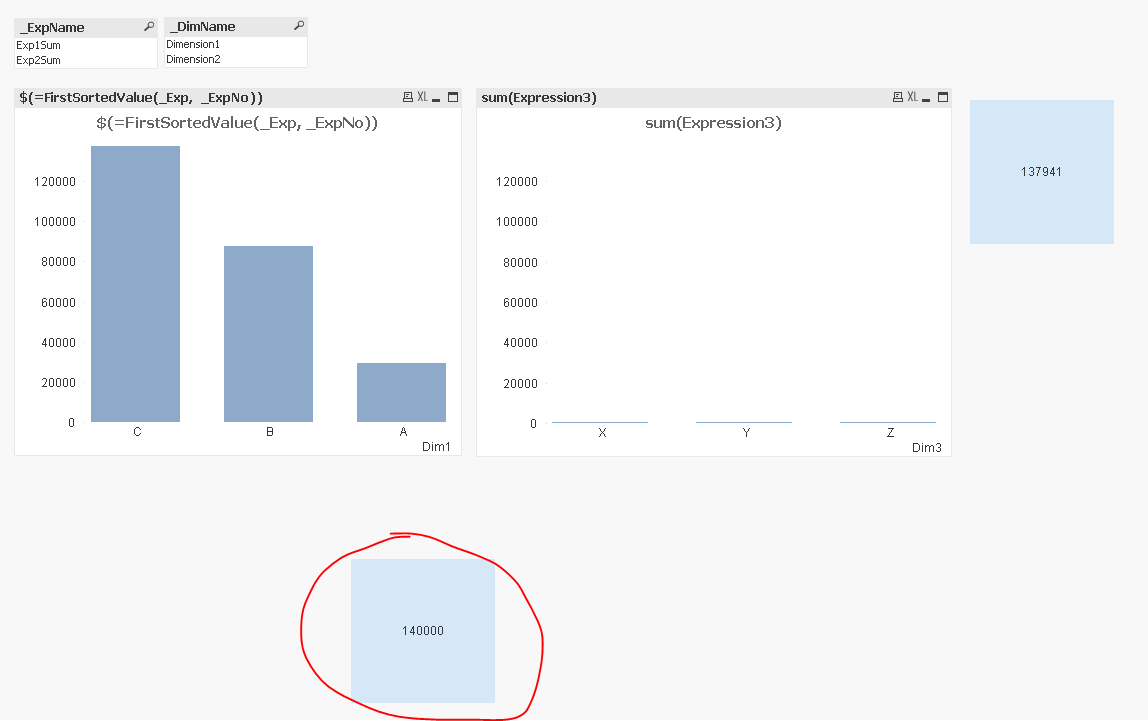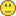Announcements
The way to achieve your own success is the willingness to help somebody else. Go for it!
cancel
Showing results for
Did you mean:Creator

## Rounding a number

Hi community,

It looks like a silly question, but I couldn't find an answer and wasn't able to work it around myself, so:

How can I dynamically round a number, so that the final value is first two digits of the number plus 1 and the rest are zeroes:

InitialAfter rounding
1234513000
123130
998710000
510

Would appreciate any help

Thanks1 Solution

Accepted SolutionsMVP

Something like this in the script

Table:

Ceil(Initial, pow(10, RangeMax(Len(Initial)-2, 1))) as [After rounding];

Initial

12345

123

9987

5

];10 RepliesMVP

Try this

=Ceil(Initial, pow(10, RangeMax(Len(Initial)-2, 1)))MVP

Something like this in the script

Table:

Ceil(Initial, pow(10, RangeMax(Len(Initial)-2, 1))) as [After rounding];

Initial

12345

123

9987

5

];Creator
Author

Thanks Sunny, that's what I was looking for, but forgot to mention, that I'm using a complex expression as Initial:

=RangeMax(

max(aggr(\$(=FirstSortedValue(_Exp, _ExpNo))), "\$(=FirstSortedValue(_DimMonthFieldName, _DimMonthNo))"),

max(aggr(\$(vAmount), [Month Field]))

)

And for some reason with the expression suggested solution does not workMVP

Would it be possible to share a sample to test this out?Creator
Author

Yeah, will do in a couple of minsCreator
Author

I've created a small app which it can be tested onMVP

This

=Ceil(RangeMax(

max(aggr(\$(=FirstSortedValue(_Exp, _ExpNo)), \$(=FirstSortedValue(_DimField, _DimNo)))),

max(aggr(sum(Expression3), Dim3))

), pow(10, RangeMax(Len(RangeMax(

max(aggr(\$(=FirstSortedValue(_Exp, _ExpNo)), \$(=FirstSortedValue(_DimField, _DimNo)))),

max(aggr(sum(Expression3), Dim3))

))-2, 1)))

gives me 140000

original value was 137941... is that not what you wanted?MVP

It even works for static maxCreator
Author

Yeahh, sure it's working, Thank you, Sunny,

I just totally forgot that Initial value can have fractional part...put floor() before rangemax and now it's workingTags
Community Browser